# NCERT Solutions for Class 10 Maths Chapter 9 - Some Applications of Trigonometry

Share

NCERT Mathematics Solutions for Class 10th Chapter 9- Some Applications of Trigonometry, Goprep is providing students with a simple and easy way to have a better understanding of the topics. Covering the complete syllabus and based on current CBSE curriculum, these Solutions help students to solve the Maths questions quickly and to score more marks in the exam. You can access these Solutions from the official website at free of cost.

Chapter 9 Some Applications of Trigonometry discusses some ways in which Trigonometry is used in the life around us. You will learn how Trigonometry is used for finding the heights and distances of various objects without measuring them. Further, get to know the astonishing facts, images, geometrical figures, and formative applications aid by using these guided NCERT Solutions.

## NCERT Solutions for Class 10 Maths Chapter 9 - Some Applications of Trigonometry

Exercise 9.1
• Exercise 9.1
Class 10th|NCERT - MathematicsChapter 9 - Some Applications of Trigonometry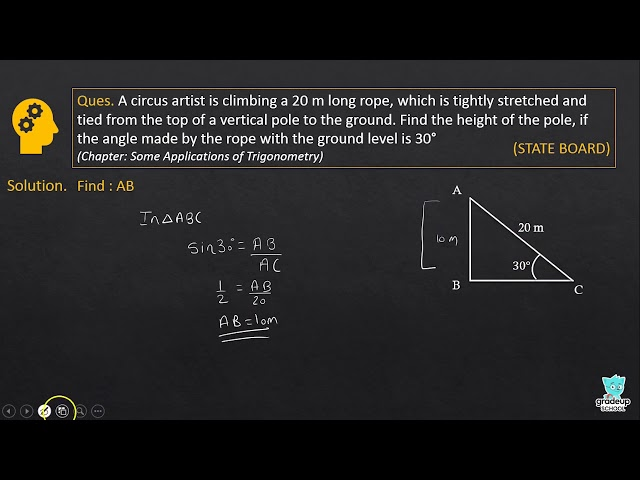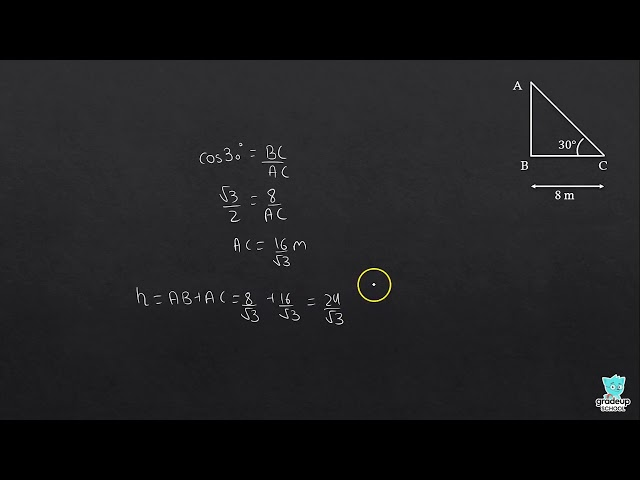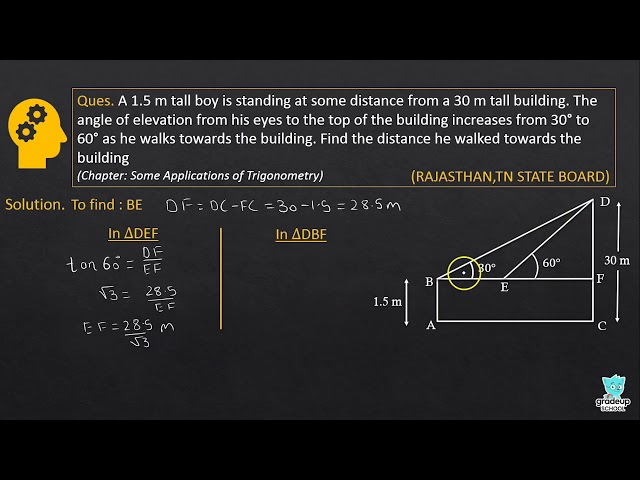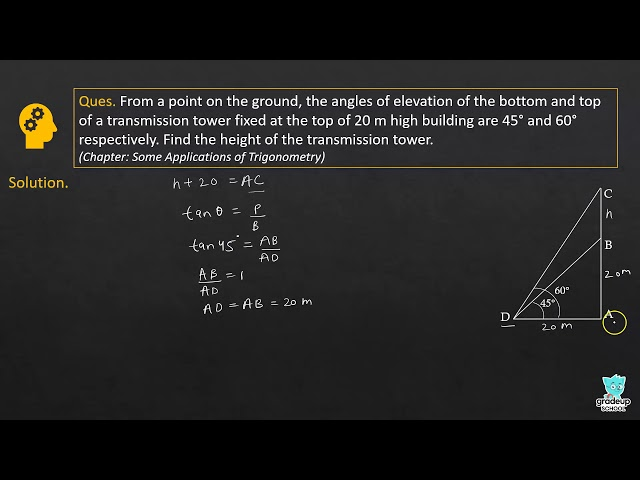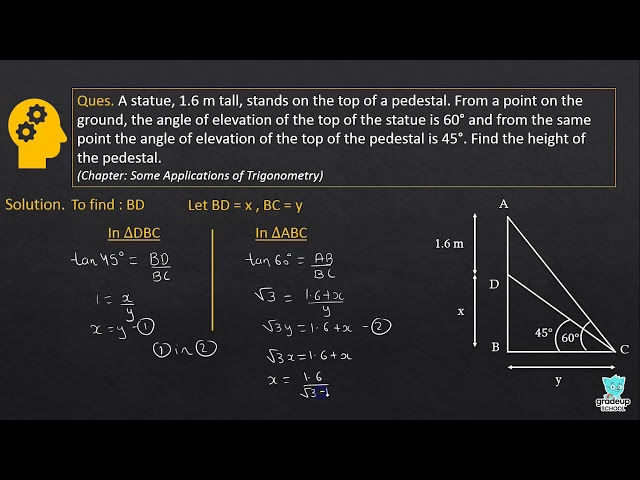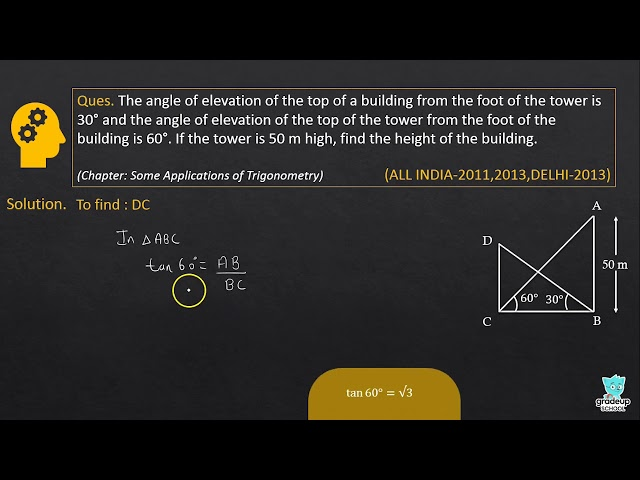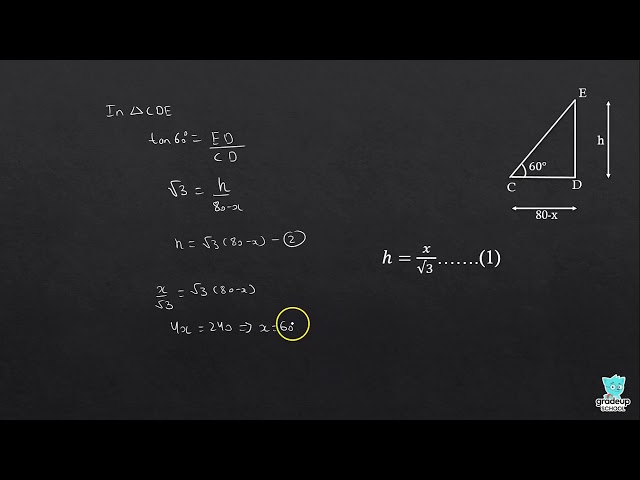See More (6)
NCERT - MathematicsClass 10th , NCERT
 Chapter 1 - Real Numbers Chapter 2 - Polynomials Chapter 3 - Pair of Linear Equations in Two Variables Chapter 4 - Quadratic Equations Chapter 5 - Arithmetic Progressions Chapter 6 - Triangles Chapter 7 - Coordinate Geometry Chapter 8 - Introduction to Trigonometry Chapter 9 - Some Applications of Trigonometry Chapter 10 - Circles Chapter 11 - Constructions Chapter 12 - Areas Related to Circles Chapter 13 - Surface Areas and Volumes Chapter 14 - Statistics Chapter 15 - Probability